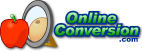## Welcome to OnlineConversion.com

Convert Miles to Meters
Convert Meters to Miles

To convert from miles to meters by hand, multiply miles by 1609.344
miles * 1609.344 = meters

To convert from meters to miles by hand, divide meters by 1609.344
meters / 1609.344 = miles

An automated version of this calculator can be found here:
Common Distance Conversions
Metric Distance Conversions
All Distance Conversions

(and now the technical stuff, if you're interested)

There are 5280 feet in a mile. Using the international definition of the foot of exactly 30.48 centimeters we find that a mile is exactly 1609.344 meters.

1 mi * 5280 ft/mi = 5280 ft
5280 ft * 30.48 cm/ft = 160934.4 cm
160934.4 cm / 100 cm/m = 1609.344 m

The word mile comes from the Latin word mille, meaning 1000, since the mile was originally 1000 paces (2000 steps). Back in the year 1592 England defined the mile to be 8 furlongs, which is 80 chains, or 320 rods, or 1760 yards, or 5280 feet. This is the basic definition we still use today, the only difference being how we define the foot.

Symbols used:
mile = mi
foot (or feet) = ft
meter = m
kilometer = km
centimeter = cm### BookMark Us

It may come in handy.

Check out our Conversion Software for Windows.Can't find something?
Try searching.

Are you bored?
Try the Fun Stuff.